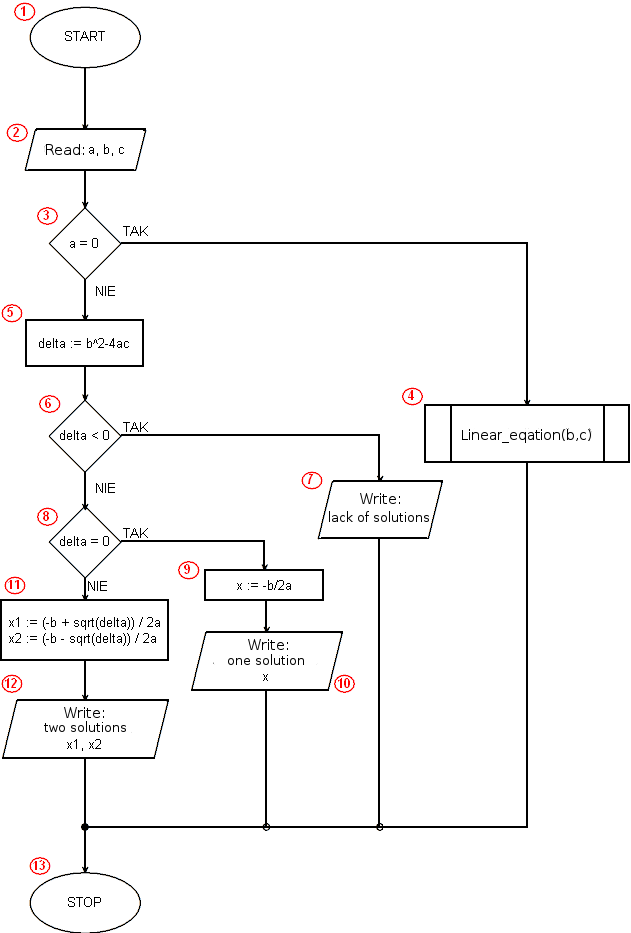# Implementation of the algorithm solving a quadratic equation in Pythonimport math

def liniowe(a,b):
if a == 0:
if b == 0:
print('identity equation')
else:
print('contrary equation')
else:
x = -b / a
print('x = ', x)

a = float(input('a = '))
b = float(input('b = '))
c = float(input('c = '))
if a == 0:
liniowe(b,c)
else:
delta = b * b - 4 * a * c
if delta < 0:
print('lack of solutions')
elif delta == 0:
x=-b / (2 * a)
print('one solution x = ', x)
else:
x1 = (-b + math.sqrt(delta)) / (2 * a)
x2 = (-b - math.sqrt(delta)) / (2 * a)
print('x1 = ', x1)
print('x2 = ', x2)

 Program line Description Algorithm block 1 We inform the Python environment that our program will use the math library. We use the sqrt() square root function defined in this library. - 3 - 11 We define a linear function that implements an algorithm for solving a linear equation. 4 13 - 15 Using the input() function, we print prompt messages to the user on the screen and load the values of the coefficients a, b and c entered by him into the appropriate variables. The float() function converts the user's input into a floating point number (float). 2 16 We check if the coefficient a is equal to 0. If so, we are dealing with a linear equation. 3 17 We will be in this line only when a = 0, i.e. when the equation is a linear equation. We call the function linear(b, c), which performs the algorithm for solving a linear equation. It is worth noting that the coefficient to the first power of x is denoted by b, and the intercept is c (traditionally, a and b are used respectively in a linear equation). 4 18 else, i.e. in the opposite case. At this point, the solution of the equation begins when a ≠ 0, i.e. when we are actually dealing with a quadratic equation. - 19 We calculate the value of the delta variable. 5 20 We check if delta < 0. 6 21 When delta < 0, we print an appropriate message about the lack of solutions. 7 22 This is where the program block starts when delta is not lower than zero, i.e. when the equation has solutions (one or two). At the same time we check if delta = 0 - elif instruction. 8 23 We calculate and save in the variable x the solution of the equation (one, double - delta = 0). 9 24 We display the calculated result. 10 25 We start the program block executed when the delta = 0 condition was not met (and the earlier delta < 0 and a ≠ 0). - 26 - 27 We calculate solutions and assign their values to variables x1 and x2. 11 28 - 29 We display the calculated result - two solutions of the quadratic equation. 12